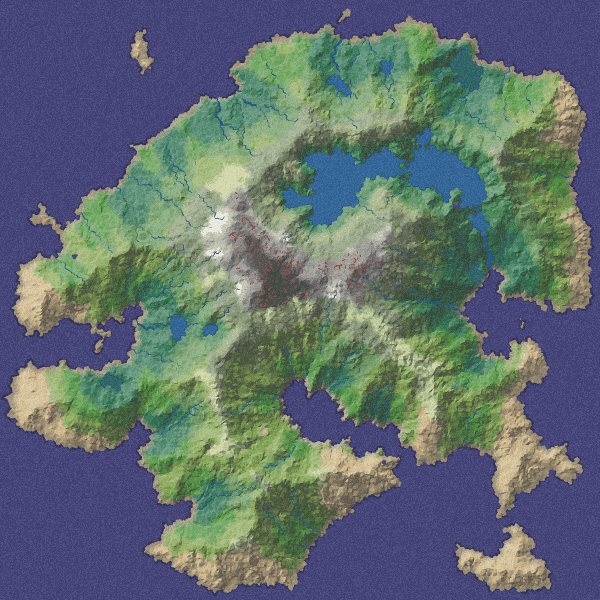# Stoat-finding particle filter¶

Our friend Data Stoat has gone missing! The GPS sensor that they normally carry has stopped working. But Data still has a low-res camera with mobile uplink, so we know what sort of scenery they're in. Can you help find Data Stoat?

Submit your answer on Moodle, in the form of a greyscale png file the same dimensions as the map. Your png should be a heatmap, where each pixel is coloured according to the probability that Data Stoat is in that location, white = highest probability, black = zero probability. I will normalize the pixels to form a proper probability distribution, and your score will be the probability you assign to Data Stoat's true location.#### Hidden Markov models¶

The underlying probability model is a hidden Markov model, where $X_n$ is the location at timestep $n$ and $Y_n$ is the noisy observation. We wish to compute the distribution of $X_n$ given observations $(y_0,\dots,y_n)$.

$$\begin{eqnarray} &X_0& \to &X_1& \to &X_2& \to \cdots\\ &\downarrow& &\downarrow& &\downarrow&\\ &Y_0& &Y_1& &Y_2& \end{eqnarray}$$

We can find this distribution iteratively. Suppose we know the distribution of $(X_{n-1} | y_0,\dots,y_{n-1})$. Then we can find the distribution of $(X_n | y_0,\dots,y_{n-1})$ using the law of total probability and memorylessness,

$$\Pr(x_n|y_0,\dots,y_{n-1}) = \sum_{x_{n-1}} \Pr(x_n |x_{n-1}) \Pr(x_{n-1}|y_0,\dots,y_{n-1})$$

and then we can find the distribution of $(X_n | y_0,\dots,y_n)$ using Bayes's rule and memorylessness,

$$\Pr(x_n|y_0,\dots,y_{n-1},y_n) = \text{const}\times\Pr(x_n|y_0,\dots,y_{n-1}) \Pr(y_n|x_n).$$

The method is laid out in Example Sheet 4, which you should attempt first, before coding. The example sheet asks you to solve the equations exactly, producing a vector of probabilities, $\Pr(x_n|y_0,\dots,y_n)$ for each location $x_n$ on the map. But when the map is large, then it's not practical to compute the sum nor the constant. Instead, we can use an empirical approximation.

#### Empirical approximation¶

The idea of empirical approximation is that, instead of working with probability distributions, we work with weighted samples, and we choose the weights so as approximate the distribution we're interested in. Formally, if we want to approximate the distribution of a random variable $Z$, and we have a collection of points $z_1,\dots,z_n$, we want weights so that $$\mathbb{P}(Z\in A) \approx \sum_{i=1}^n w_i 1_{z_i\in A}.$$ We've used weighted samples in two ways in the course:

• For the simple Monte Carlo approximation, we sample $z_i$ from $Z$, and we let all the weights be the same, $1/n$.

• For computational Bayes's rule, we sample $z_i$ from the prior distribution, and let the weights be proportional to the likelihood of the data conditional on $z_i$.

This practical will use an empirical approximation technique called a particle filter. In the particle filter, we'll use weighted samples to approximate the distributions of $(X_n | y_0,\dots,y_{n-1})$ and of $(X_n|y_0,\dots,y_n)$. Each sample is called a particle.

## Data import¶

We have data from several animals which are wandering over a terrain. The animals are equipped with GPS and with cameras, but the GPS on animal 0 has stopped working. We would like to find out where this animal is.

Here is the terrain and the GPS+camera data. The camera records roughly the rgb values of the animal's current location, though there is some noise.

Coding note. Given an image map_image, we can treat it as a simple array, and get at the pixels with map_image[i,j]. I'll interpret this as x-coordinate i and y-coordinate j. However, the plotting routine ax.imshow interprets the first coordinate as y and the second as x, so I need to transpose the array before plotting it.

## 0. Empirical approximation of $X_0$¶

We'll start with a prior belief that the animal's location $X_0$ is uniformly distributed over the map.

The first step is to create a sample from this initial distribution. We'll store it as an $M\times 3$ array, one row per particle, with columns for $x$ coordinate, $y$ coordinate, and weight $w$. The prior distribution is uniform, so $w=1/M$. We'll call this matrix δ0.

Here's also a handy function to visualize the particles, use show_particles.

## 1. Empirical approximation of $(X_0|y_0)$: updated weights¶

Let's first find the distribution of $(X_0|y_0)$, where $y_0$ is the first observation. Bayes's rule tells us the distribution:

$$\operatorname{Pr}_{X_0}(x|y_0)=\text{const}\times \operatorname{Pr}_{X_0}(x)\Pr(y_0|X_0=x).$$

We've seen the empirical approximation for this, in lecture notes on computational Bayes's rule. We take a sample from $X_0$, and we set the weight of sample point $p$ to be proportional to $\Pr(y_0|X_0=p)$. We already build ourselves a sample from $X_0$, in the previous section. We'll call our new weighted particles π0.

To apply Bayes's rule, we need a probability model for $Y_0$ given a particle's location. A reasonable guess is that $Y_0$ is a noisy version of the colour patch around the supposed location. Here's a handy utility to extract the average colour of a patch:

Implement a pr(y,loc) function to compute the probability of observing y if the true location is loc.
It's up to you to invent the probability model. A reasonable probability model is that the observed rgb values in y are independent Gaussian random variables, with mean patch(map_image, loc), and with a standard deviation that you should pick. If you want to be cleverer, consider a probability model based on closeness in HSL colour space.
Now we can set the weight of all the particles, and thereby obtain a sample of $(X_0|y_0)$.